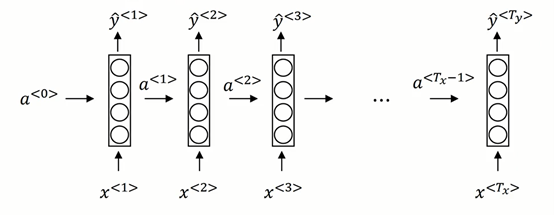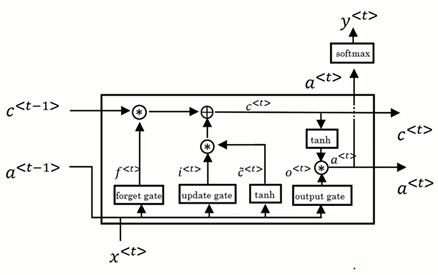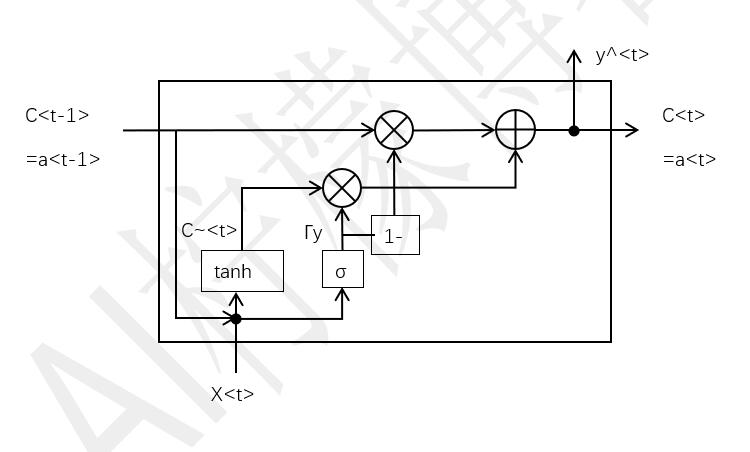# 一文看懂循环神经网络基本原理

(在苹果系统下，如果文章中的图片不能正常显示，请升级Safari浏览器到最新版本，或者使用Chrome、Firefox浏览器打开。)

RNN是循环神经网络的缩写，并且也是循环网络结构中的一种，我们通常使用这种网络模型来处理序列型的数据。语音识别处理的就是一个典型的有时间顺序的序列数据，自然语言文本也是。在一个普通的DNN网络中，层与层之间是全连接的，而每层中的神经元节点之间不存在任何连接，这样的一种普通DNN网络结构难以解决很多问题。以语音识别为例，不同时刻t的语音包含的字，在推理计算时，需要根据上下文来确定应该输出为什么字符，而且结果应当跟具体所在时刻t无关，否则会出现不同时间说相同的字会产生不同的识别输出的问题。

循环网络就解决了这个问题，这有点类似于隐马尔可夫模型，对于每一时刻的输入，所产生的输出值，不仅仅依赖于当前时刻t，还依赖于前N个时刻的输出值。这主要是通过在每一个循环层单元中，添加了一个记忆单元实现的。图1 一个普通循环层原理图

令初始向量a<0>=0，对于每一个t时刻，循环层的值a<t>和y^<t>计算公式为

–$$a^{<t>}=g(w_{aa} a^{<t-1>}+w_{ax} x^{<t>}+b_a) \tag{1}$$

–$$\hat{y}^{<t>}=g(w_{ya}*a^{<t>}+b_y) \tag{2}$$图2 沿时间反向传播原理图

与此同时，常用的循环结构还有LSTM和GRU等，分别称为长短时记忆网络和门控循环单元。这两种循环层对于捕捉深层连接、解决诸如梯度消失，以及更长时间范围的上下文依赖，有着更好的效果。图3 一个LSTM单元

$$\gamma_\mu=\sigma(W_c [a^{<t-1>},x^{<t>} ]+b_\mu) \tag{4}$$

$$a^{<t>}=\gamma_o*tanh⁡ (c^{<t>}) \tag{7}$$

$$y^{<t>}=g(a^{<t>}) \tag{9}$$图4 GRU单元数据流图

GRU单元结构将遗忘门和输入门合并为一个更新门，从而减少了参数数量，降低了计算复杂度，从而以利于加深模型。这种结构的循环层单元逐渐流行起来。

$$\widetilde{c}^{<t>}=tanh⁡(w_c [c^{<t-1>},x^{<t>} ]+b_c) \tag{10}$$
$$\gamma_y=\sigma⁡(w_y [c^{<t-1>},x^{<t>} ]+b_y) \tag{11}$$
$$c^{<t>}=\gamma_y * \widetilde{c}^{<t>}+(1-\gamma_y )*c^{<t-1>} \tag{12}$$
$$a^{<t>}=c^{<t>} \tag{13}$$
$$y^{<t>}=g(a^{<t>}) \tag{14}$$

 版权声明本博客的文章除特别说明外均为原创，本人版权所有。欢迎转载，转载请注明作者及来源链接，谢谢。本文地址: https://blog.ailemon.me/2020/10/19/rnn-theory/ All articles are under Attribution-NonCommercial-ShareAlike 4.0